Next: Induced Flow Up: Incompressible Aerodynamics Previous: Zhukovskii's Hypothesis

# Vortex Sheets

A vortex sheet is defined as a planar array of parallel vortex filaments. Consider a uniform vortex sheet, lying in the-plane, in which the vortex filaments run parallel to the-axis. (See Figure 9.7.) The vorticity within the sheet can be written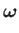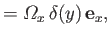(9.54)

where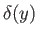is a Dirac delta function. Here,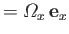is the sheet's vortex intensity per unit length. Let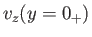and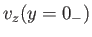be the-component of the fluid velocity immediately above and below the sheet, respectively. Consider a small rectangular loop in the-plane that straddles the sheet, as shown in the figure. Integration of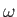around the loop (making use of the curl theorem) yields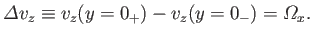(9.55)

In other words, a vortex sheet induces a discontinuity in the tangential flow across the sheet. The previous expression can easily be generalized to give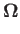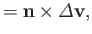(9.56)

whereis the sheet's vortex intensity per unit length,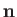is a unit vector normal to the sheet, and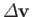is the jump in tangential velocity across the sheet (traveling in the direction of). Furthermore, it is reasonable to assume that the previous relation holds locally for non-planar and non-uniform vortex sheets.Next: Induced Flow Up: Incompressible Aerodynamics Previous: Zhukovskii's Hypothesis
Richard Fitzpatrick 2016-03-31